# Liouville theorems

(diff) ← Older revision | Latest revision (diff) | Newer revision → (diff)

Liouville's theorem on bounded entire analytic functions: If an entire functionof the complex variableis bounded, that is,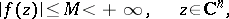thenis a constant. This proposition, which is one of the fundamental results in the theory of analytic functions, was apparently first published in 1844 by A.L. Cauchy

for the case; J. Liouville presented it in his lectures in 1847, and this is how the name arose.

Liouville's theorem can be generalized in various directions. For example, ifis an entire function inandfor some integer, thenis a polynomial in the variables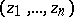of degree not exceeding. Moreover, if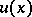is a real-valued harmonic function in the number space,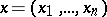, and, thenis a harmonic polynomial inof degree not exceeding(see also ).

Liouville's theorem on conformal mapping: Every conformal mapping of a domain in a Euclidean space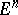withcan be represented as a finite number of compositions of very simple mappings of four kinds — translation, similarity, orthogonal transformation, and inversion. It was proved by J. Liouville in 1850 (see , Appendix 6).

This Liouville theorem shows the poverty of the class of conformal mappings in space, and from this point of view it is very important in the theory of analytic functions of several complex variables and in the theory of quasi-conformal mapping.

How to Cite This Entry:
Liouville theorems. Encyclopedia of Mathematics. URL: http://encyclopediaofmath.org/index.php?title=Liouville_theorems&oldid=19033
This article was adapted from an original article by E.D. Solomentsev, S.A. Stepanov, I.A. Kvasnikov (originator), which appeared in Encyclopedia of Mathematics - ISBN 1402006098. See original article